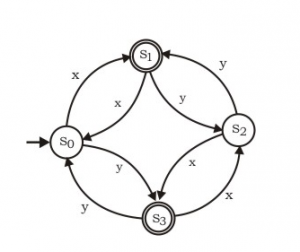GFG App
Open AppBrowser
Continue

# GATE | Gate IT 2007 | Question 47

Consider the following DFA in which s0 is the start state and s1, s3 are the final states.What language does this DFA recognize ?
(A) All strings of x and y
(B) All strings of x and y which have either even number of x and even number of y or odd number or x and odd number of y
(C) All strings of x and y which have equal number of x and y
(D) All strings of x and y with either even number of x and odd number of y or odd number of x and even number of y

Explanation:
Alphabet x would move to S1 and alphabet y would move to S3 from S0 state. Hence smallest string accepted by the DFA are {x, y}. Further possible movements from S1 or S3 to the accepting states can be any combination of x and y of even length i.e. either of {xx, xy, yx, yy} and their combinations. Hence the language accepted by the DFA can be recognized as (x+y) + (xx+xy+yx+yy)* i.e. all strings of x and y with odd length (even no. of x and odd no. of y OR even no. of y and odd no. of x).

Option elimination method: (option which has at least one example which is not accepted by DFA)
Option (A): False as {xx, xy etc} are not accepted by the DFA.
Option (B): False as even no. of x and even no. of y string {xxyy} is not accepted by the DFA.
Option (C): False as string with equal no. of x and equal no. of y {xy} is not accepted by the DFA.
Option (D): All strings with either even number of x and odd number of y or odd number of x and even number of y are accepted.
Hence the answer is option (D).

This solution is contributed by Yashika Arora

Quiz of this Question

My Personal Notes arrow_drop_up